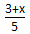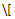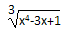# 在网页中显示数学公式

## 一．计算机表现数学公式的几种方法

### 1．面向桌面的TeX系统

TeX(http://zh.wikipedia.org/wiki/TeX)是Knuth教授开发的一种优秀的桌面电子排版系统。它提供了一套功能强大并且十分灵活的排版语言，有多达900多条指令，并且具有宏功能，用户可以不断地定义自己适用的新命令来扩展TeX系统的功能。

TeX系统有许多优点，如开源、易移植、排版质量高、输出结果与设备无关等。同时，它也是公认的最好的数学公式排版系统，它在数学和工程领域得到了广泛的使用，相关领域的学术论文基本都由TeX系统或其兼容系统（如LaTeX系统）排版生成。\frac{3+x}{5}\]
f（x）＝x2－1f(x)=x^2-1\]\sqrt{x^4-3x+1}\]

### 2．面向互联网的数学标记语言MathML

TeX系统虽然能完美地显示数学公式，但是无法在互联网上使用。目前我采用的方法是将Word中的公式进行截图，在网页中用图像的方式显示公式，用这种方法比较费时，而且修改也不方便。而HTML超文本标记语言由于自身的缺陷，也很难显示数学公式。

$<mroot> <mi>a</mi> <mi>n</mi> </mroot>$
<apply>
<root/><degree><ci type="integer">n</ci>
</degree><ci>a</ci></apply>

### 3．ASCIIMathML转换方法

ASCIIMathML(http://www1.chapman.edu/~jipsen/mathml/asciimath.html)转换程序由美国加州查普曼（Chapman）大学Peter Jipsen开发，其设计思想是在网页上插入一段JS代码，将网页中的TeX指令（TeX/LaTeX-style）自动转换成MathML表现标记语言，再返回给支持MathML标准的网络浏览器识别和显示。

### 4．其他方法

<img src="http://latex.codecogs.com/gif.latex?\sqrt{a^2+b^2}" title="\sqrt{a^2+b^2}" />

## 二、在Web系统中显示和输入数学公式

<script type="text/javascript" src="http://cdn.mathjax.org/mathjax/latest/MathJax.js?config=TeX-AMS-MML_HTMLorMML"></script>